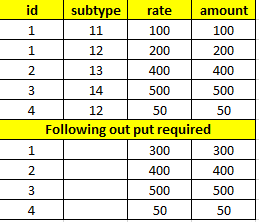# Case statement for sum in mysql querry

I have following mysql table as attached in image below. and here is my working query. There are two column which need to be sum on the basis of group by. (Rate and Amount). Currently everything is working fine, but I want to knew is there any way by which I can write just one case statement as it apply on all column which need to be sum ??? is this possible ???

``````   SELECT id, SUM(CASE WHEN subtype in (11, 12) THEN rate ELSE rate END) as ratesum,
SUM(CASE WHEN subtype in (11, 12) THEN Amount ELSE rate END) as amountsum
from test1 GROUP BY id;
``````So what happens if you have this? What’s the output?

``````ID  SubType        Rate  Amount
--- -------        ----  ------
1     11           100    100
1     12           200    200
1     13           400    200
``````

out is absolutely fine. but i want to knew can i use one case statement for all column instead of case statement with every column

No, because case statements return a single value, not an array/list. So you need one for each column.

1 Like

ok thanks alot sir!